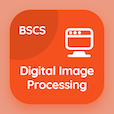Computer Science Courses Online

Chapter 11: Database Management System Practice Tests

Database Management System MCQs - Chapter 11

# Relational Algebra and Calculus Multiple Choice Questions (MCQ Quiz) PDF - 1

The Book Relational Algebra and Calculus Multiple Choice Questions and Answers (MCQs) PDF, Relational Algebra and Calculus quiz answers, test 1 to study online Database Management System Course. Practice SELECT and PROJECT MCQs, Relational Algebra and Calculus trivia questions and answers PDF to prepare for job interview. The eBook Relational Algebra and Calculus MCQs App Download: binary relational operation: join and division, tuple relational calculus, division operation career test for online college classes.

The Multiple Choice Question (MCQ Quiz): In unary relational operations, the SELECT operation is partition of relation usually classified as PDF, Relational Algebra and Calculus App Download (Free) with vertical partition, horizontal partition, insert partition, and delete partition choices for bachelor's degree in computer science. Solve select and project quiz questions, download Google eBook (Free Sample) for online computer science and engineering.

## Database Management System: Relational Algebra & Calculus MCQs Questions

MCQ: In unary relational operations, the SELECT operation is partition of relation usually classified as

A) horizontal partition
B) vertical partition
C) insert partition
D) delete partition

MCQ: The operators in SELECT operation statement such as 'and' , 'or' and 'not' are classified as

A) Boolean operators
B) tuple operators
C) string operators
D) insertion operators

MCQ: If in the JOIN operation, the conditions of JOIN operation are not satisfied then the results of the operation is

A) zero tuples and empty relation
B) one tuple from one relation
C) zero tuples from two relation
D) two tuples from empty relations

MCQ: Because of the calculus expression, the relational calculus is considered as

A) procedural language
B) non procedural language
C) structural language
D) functional language

MCQ: The DIVISION operation can be applied to two relations A and B such as R(A) ÷ R(B) where as

A) A does not belong to B
B) A must be added to B
C) A belongs or equal to B
D) A must be subtracted from B

Download Database Management System Quiz App, Computer Networks MCQ App, and Digital Image Processing MCQs App to install for Android & iOS devices. These Apps include complete analytics of real time attempts with interactive assessments. Download Play Store & App Store Apps & Enjoy 100% functionality with subscriptions!